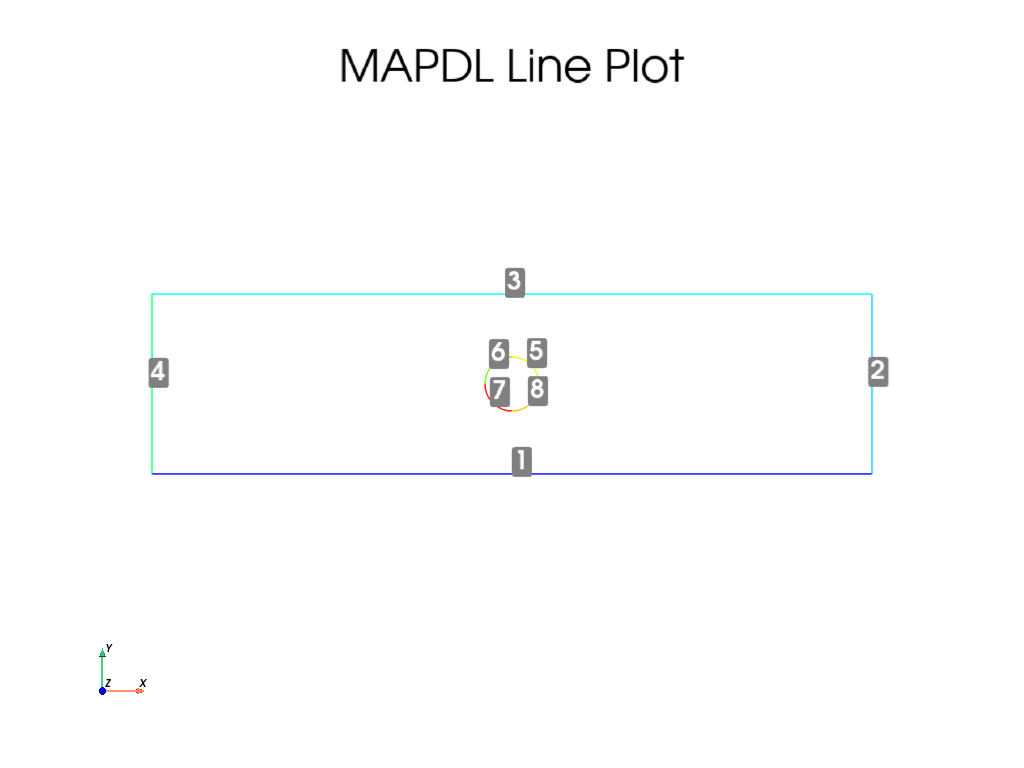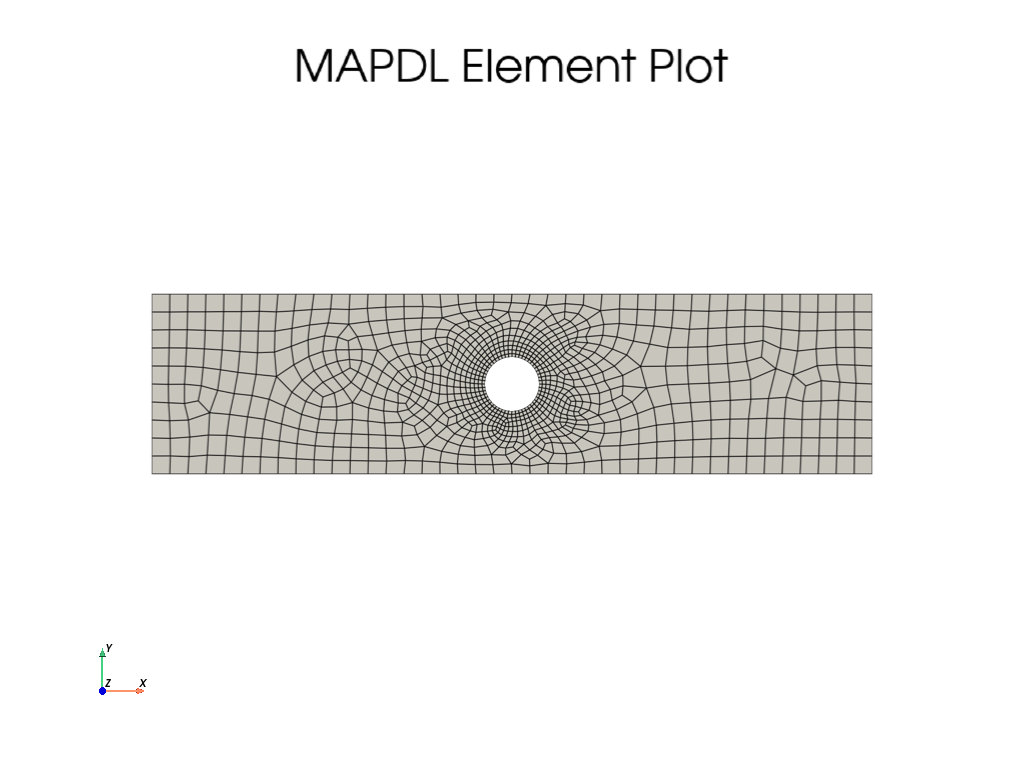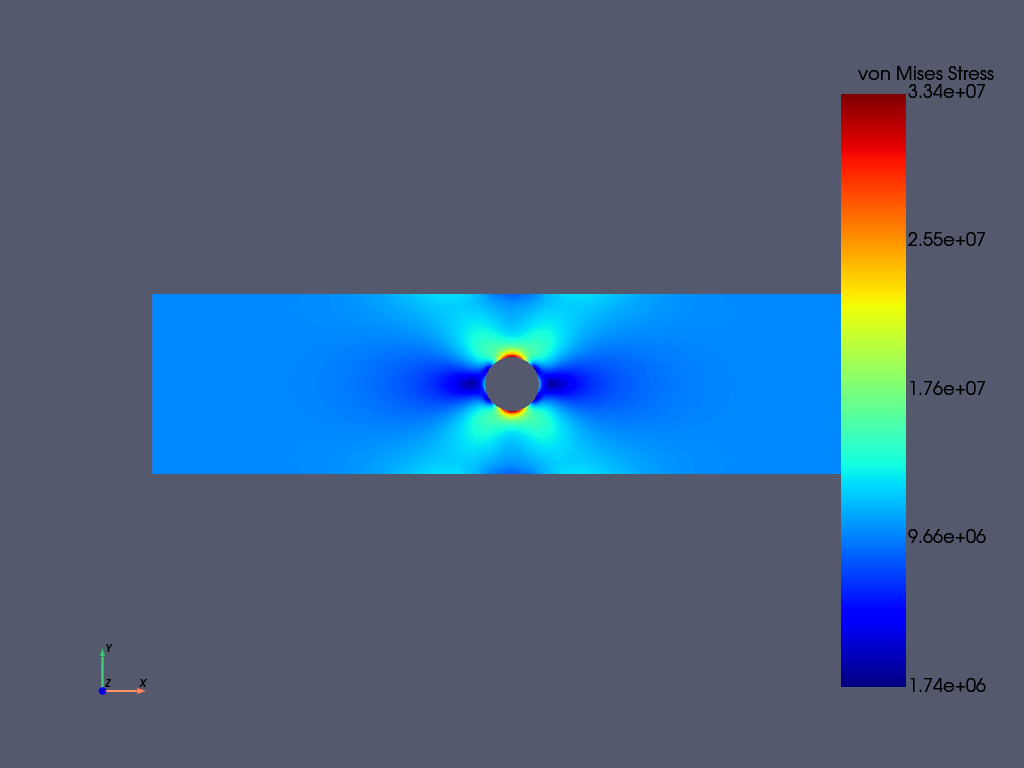# MAPDL 2D Plane Stress Concentration Analysis#

This tutorial shows how you can use PyMAPDL to determine and verify the “stress concentration factor” when modeling using 2D plane elements and then verify this using 3D elements.

First, start MAPDL as a service.

# sphinx_gallery_thumbnail_number = 3

import matplotlib.pyplot as plt
import numpy as np

from ansys.mapdl.core import launch_mapdl

mapdl = launch_mapdl()


## Element Type and Material Properties#

This example will use PLANE183 elements as a thin plate can be modeled with plane elements provided that KEYOPTION 3 is set to 3 and a thickness is provided.

This example will use SI units.

mapdl.prep7()
mapdl.units("SI")  # SI - International system (m, kg, s, K).

# define a PLANE183 element type with thickness
mapdl.et(1, "PLANE183", kop3=3)
mapdl.r(1, 0.001)  # thickness of 0.001 meters)

# Define a material (nominal steel in SI)
mapdl.mp("EX", 1, 210e9)  # Elastic moduli in Pa (kg/(m*s**2))
mapdl.mp("DENS", 1, 7800)  # Density in kg/m3
mapdl.mp("NUXY", 1, 0.3)  # Poisson's Ratio

# list currently defined material properties
print(mapdl.mplist())

LIST MATERIALS        1 TO        1 BY        1
PROPERTY= ALL

MATERIAL NUMBER        1

TEMP        EX
0.2100000E+12

TEMP        NUXY
0.3000000

TEMP        DENS
7800.000


## Geometry#

Create a rectangular area with the hole in the middle. To correctly approximate an infinite plate, the maximum stress must occur far away from the edges of the plate. A length to width factor can approximate this.

length = 0.4
width = 0.1

ratio = 0.3  # diameter/width
diameter = width * ratio

# create the rectangle
rect_anum = mapdl.blc4(width=length, height=width)

# create a circle in the middle of the rectangle
circ_anum = mapdl.cyl4(length / 2, width / 2, radius)

# Note how pymapdl parses the output and returns the area numbers
# created by each command.  This can be used to execute a boolean
# operation on these areas to cut the circle out of the rectangle.
plate_with_hole_anum = mapdl.asba(rect_anum, circ_anum)

# finally, plot the lines of the plate
mapdl.lplot(cpos="xy", line_width=3, font_size=26, color_lines=True, background="w")## Meshing#

Mesh the plate using a higher density near the hole and a lower density for the remainder of the plate by setting LESIZE for the lines nearby the hole and ESIZE for the mesh global size.

Line numbers can be identified through inspection using lplot

# ensure there are at 50 elements around the hole
hole_esize = np.pi * diameter / 50  # 0.0002
plate_esize = 0.01

# increased the density of the mesh at the center
mapdl.lsel("S", "LINE", vmin=5, vmax=8)
mapdl.lesize("ALL", hole_esize, kforc=1)
mapdl.lsel("ALL")

# Decrease the area mesh expansion.  This ensures that the mesh
# remains fine nearby the hole
mapdl.mopt("EXPND", 0.7)  # default 1

mapdl.esize(plate_esize)
mapdl.amesh(plate_with_hole_anum)
mapdl.eplot(
vtk=True,
cpos="xy",
show_edges=True,
show_axes=False,
line_width=2,
background="w",
)## Boundary Conditions#

Fix the left-hand side of the plate in the X direction and set a force of 1 kN in the positive X direction.

# Fix the left-hand side.
mapdl.nsel("S", "LOC", "X", 0)
mapdl.d("ALL", "UX")

# Fix a single node on the left-hand side of the plate in the Y
# direction.  Otherwise, the mesh would be allowed to move in the y
# direction and would be an improperly constrained mesh.
mapdl.nsel("R", "LOC", "Y", width / 2)
assert mapdl.mesh.n_node == 1
mapdl.d("ALL", "UY")

# Apply a force on the right-hand side of the plate.  For this
# example, we select the nodes at the right-most side of the plate.
mapdl.nsel("S", "LOC", "X", length)

# Verify that only the nodes at length have been selected:
assert np.allclose(mapdl.mesh.nodes[:, 0], length)

# Next, couple the DOF for these nodes.  This lets us provide a force
# to one node that will be spread throughout all nodes in this coupled
# set.
mapdl.cp(5, "UX", "ALL")

# Select a single node in this set and apply a force to it
# We use "R" to re-select from the current node group
mapdl.nsel("R", "LOC", "Y", width / 2)
mapdl.f("ALL", "FX", 1000)

# finally, be sure to select all nodes again to solve the entire solution
mapdl.allsel(mute=True)


## Solve the Static Problem#

Solve the static analysis

mapdl.run("/SOLU")
mapdl.antype("STATIC")
output = mapdl.solve()
print(output)

*****  MAPDL SOLVE    COMMAND  *****

*** NOTE ***                            CP =       1.304   TIME= 08:27:02
There is no title defined for this analysis.

*** SELECTION OF ELEMENT TECHNOLOGIES FOR APPLICABLE ELEMENTS ***
---GIVE SUGGESTIONS ONLY---

ELEMENT TYPE         1 IS PLANE183 WITH PLANE STRESS OPTION. NO SUGGESTION IS
AVAILABLE.

*** MAPDL - ENGINEERING ANALYSIS SYSTEM  RELEASE 2023 R1          23.1     ***
Ansys Mechanical Enterprise
00000000  VERSION=LINUX x64     08:27:02  JUN 02, 2023 CP=      1.328

S O L U T I O N   O P T I O N S

PROBLEM DIMENSIONALITY. . . . . . . . . . . . .2-D
DEGREES OF FREEDOM. . . . . . UX   UY
ANALYSIS TYPE . . . . . . . . . . . . . . . . .STATIC (STEADY-STATE)
GLOBALLY ASSEMBLED MATRIX . . . . . . . . . . .SYMMETRIC

*** NOTE ***                            CP =       1.337   TIME= 08:27:02
Present time 0 is less than or equal to the previous time.  Time will
default to 1.

*** NOTE ***                            CP =       1.343   TIME= 08:27:02
The conditions for direct assembly have been met.  No .emat or .erot
files will be produced.

L O A D   S T E P   O P T I O N S

LOAD STEP NUMBER. . . . . . . . . . . . . . . .     1
TIME AT END OF THE LOAD STEP. . . . . . . . . .  1.0000
NUMBER OF SUBSTEPS. . . . . . . . . . . . . . .     1
STEP CHANGE BOUNDARY CONDITIONS . . . . . . . .    NO
PRINT OUTPUT CONTROLS . . . . . . . . . . . . .NO PRINTOUT
DATABASE OUTPUT CONTROLS. . . . . . . . . . . .ALL DATA WRITTEN
FOR THE LAST SUBSTEP

SOLUTION MONITORING INFO IS WRITTEN TO FILE= file.mntr

**** CENTER OF MASS, MASS, AND MASS MOMENTS OF INERTIA ****

CALCULATIONS ASSUME ELEMENT MASS AT ELEMENT CENTROID

TOTAL MASS =  0.30649

MOM. OF INERTIA         MOM. OF INERTIA

XC =  0.20000          IXX =   0.1024E-02      IXX =   0.2576E-03
YC =  0.49997E-01      IYY =   0.1642E-01      IYY =   0.4156E-02
ZC =   0.0000          IZZ =   0.1744E-01      IZZ =   0.4414E-02
IXY =  -0.3065E-02      IXY =   0.8905E-09
IYZ =    0.000          IYZ =    0.000
IZX =    0.000          IZX =    0.000

*** MASS SUMMARY BY ELEMENT TYPE ***

TYPE      MASS
1  0.306487

Range of element maximum matrix coefficients in global coordinates
Maximum = 1.265116826E+09 at element 67.
Minimum = 359465553 at element 773.

*** ELEMENT MATRIX FORMULATION TIMES
TYPE    NUMBER   ENAME      TOTAL CP  AVE CP

1       977  PLANE183      0.107   0.000109
Time at end of element matrix formulation CP = 1.49750602.

SPARSE MATRIX DIRECT SOLVER.
Number of equations =        6124,    Maximum wavefront =     48
Memory allocated for solver              =     8.435 MB
Memory required for in-core solution     =     8.134 MB
Memory required for out-of-core solution =     4.318 MB

*** NOTE ***                            CP =       1.634   TIME= 08:27:02
The Sparse Matrix Solver is currently running in the in-core memory
mode.  This memory mode uses the most amount of memory in order to
avoid using the hard drive as much as possible, which most often
results in the fastest solution time.  This mode is recommended if
enough physical memory is present to accommodate all of the solver
data.
Sparse solver maximum pivot= 1.958386732E+09 at node 1937 UY.
Sparse solver minimum pivot= 3839644.71 at node 960 UY.
Sparse solver minimum pivot in absolute value= 3839644.71 at node 960
UY.

*** ELEMENT RESULT CALCULATION TIMES
TYPE    NUMBER   ENAME      TOTAL CP  AVE CP

1       977  PLANE183      0.153   0.000157

TYPE    NUMBER   ENAME      TOTAL CP  AVE CP

1       977  PLANE183      0.051   0.000052
*** LOAD STEP     1   SUBSTEP     1  COMPLETED.    CUM ITER =      1
*** TIME =   1.00000         TIME INC =   1.00000      NEW TRIANG MATRIX

*** MAPDL BINARY FILE STATISTICS
BUFFER SIZE USED= 16384
1.562 MB WRITTEN ON ASSEMBLED MATRIX FILE: file.full
1.000 MB WRITTEN ON RESULTS FILE: file.rst


## Post-Processing#

The static result can be post-processed both within MAPDL and outside of MAPDL using pyansys. This example shows how to extract the von Mises stress and plot it using the pyansys result reader.

# grab the result from the mapdl instance
result = mapdl.result
result.plot_principal_nodal_stress(
0,
"SEQV",
lighting=False,
cpos="xy",
background="w",
text_color="k",
)

nnum, stress = result.principal_nodal_stress(0)
von_mises = stress[:, -1]  # von-Mises stress is the right most column

# Must use nanmax as stress is not computed at mid-side nodes
max_stress = np.nanmax(von_mises)## Compute the Stress Concentration#

The stress concentration $$K_t$$ is the ratio of the maximum stress at the hole to the far-field stress, or the mean cross sectional stress at a point far from the hole. Analytically, this can be computed with:

$$\sigma_{nom} = \frac{F}{wt}$$

Where

• $$F$$ is the force

• $$w$$ is the width of the plate

• $$t$$ is the thickness of the plate.

Experimentally, this is computed by taking the mean of the nodes at the right-most side of the plate.

# We use nanmean here because mid-side nodes have no stress
mask = result.mesh.nodes[:, 0] == length
print("Far field von mises stress: %e" % far_field_stress)
# Which almost exactly equals the analytical value of 10000000.0 Pa

Far field von mises stress: 9.999966e+06


Since the expected nominal stress across the cross section of the hole will increase as the size of the hole increases, regardless of the stress concentration, the stress must be adjusted to arrive at the correct stress. This stress is adjusted by the ratio of the width over the modified cross section width.

adj = width / (width - diameter)

# The stress concentration is then simply the maximum stress divided
# by the adjusted far-field stress.
print("Stress Concentration: %.2f" % stress_con)

Stress Concentration: 2.34


## Batch Analysis#

The above script can be placed within a function to compute the stress concentration for a variety of hole diameters. For each batch, MAPDL is reset and the geometry is generated from scratch.

def compute_stress_con(ratio):
"""Compute the stress concentration for plate with a hole loaded
with a uniaxial force.
"""
mapdl.clear("NOSTART")
mapdl.prep7()
mapdl.units("SI")  # SI - International system (m, kg, s, K).

# define a PLANE183 element type with thickness
mapdl.et(1, "PLANE183", kop3=3)
mapdl.r(1, 0.001)  # thickness of 0.001 meters)

# Define a material (nominal steel in SI)
mapdl.mp("EX", 1, 210e9)  # Elastic moduli in Pa (kg/(m*s**2))
mapdl.mp("DENS", 1, 7800)  # Density in kg/m3
mapdl.mp("NUXY", 1, 0.3)  # Poisson's Ratio
mapdl.emodif("ALL", "MAT", 1)

# Geometry
# ~~~~~~~~
# Create a rectangular area with the hole in the middle
diameter = width * ratio

# create the rectangle
rect_anum = mapdl.blc4(width=length, height=width)

# create a circle in the middle of the rectangle
circ_anum = mapdl.cyl4(length / 2, width / 2, radius)

# Note how pyansys parses the output and returns the area numbers
# created by each command.  This can be used to execute a boolean
# operation on these areas to cut the circle out of the rectangle.
plate_with_hole_anum = mapdl.asba(rect_anum, circ_anum)

# Meshing
# ~~~~~~~
# Mesh the plate using a higher density near the hole and a lower
# density for the remainder of the plate

mapdl.aclear("all")

# ensure there are at least 100 elements around the hole
hole_esize = np.pi * diameter / 100  # 0.0002
plate_esize = 0.01

# increased the density of the mesh at the center
mapdl.lsel("S", "LINE", vmin=5, vmax=8)
mapdl.lesize("ALL", hole_esize, kforc=1)
mapdl.lsel("ALL")

# Decrease the area mesh expansion.  This ensures that the mesh
# remains fine nearby the hole
mapdl.mopt("EXPND", 0.7)  # default 1

mapdl.esize(plate_esize)
mapdl.amesh(plate_with_hole_anum)

###############################################################################
# Boundary Conditions
# ~~~~~~~~~~~~~~~~~~~
# Fix the left-hand side of the plate in the X direction
mapdl.nsel("S", "LOC", "X", 0)
mapdl.d("ALL", "UX")

# Fix a single node on the left-hand side of the plate in the Y direction
mapdl.nsel("R", "LOC", "Y", width / 2)
assert mapdl.mesh.n_node == 1
mapdl.d("ALL", "UY")

# Apply a force on the right-hand side of the plate.  For this
# example, we select the right-hand side of the plate.
mapdl.nsel("S", "LOC", "X", length)

# Next, couple the DOF for these nodes
mapdl.cp(5, "UX", "ALL")

# Again, select a single node in this set and apply a force to it
mapdl.nsel("r", "loc", "y", width / 2)
mapdl.f("ALL", "FX", 1000)

# finally, be sure to select all nodes again to solve the entire solution
mapdl.allsel()

# Solve the Static Problem
# ~~~~~~~~~~~~~~~~~~~~~~~~
mapdl.run("/SOLU")
mapdl.antype("STATIC")
mapdl.solve()

# Post-Processing
# ~~~~~~~~~~~~~~~
# grab the stress from the result
result = mapdl.result
nnum, stress = result.principal_nodal_stress(0)
von_mises = stress[:, -1]
max_stress = np.nanmax(von_mises)

# compare to the "far field" stress by getting the mean value of the
# stress at the wall
mask = result.mesh.nodes[:, 0] == length

# adjust by the cross sectional area at the hole
adj = width / (width - diameter)

# finally, compute the stress concentration


Run the batch and record the stress concentration

k_t_exp = []
ratios = np.linspace(0.01, 0.5, 10)
print("    Ratio  : Stress Concentration (K_t)")
for ratio in ratios:
stress_con = compute_stress_con(ratio)
print("%10.4f : %10.4f" % (ratio, stress_con))
k_t_exp.append(stress_con)

Ratio  : Stress Concentration (K_t)
0.0100 :     2.9660
0.0644 :     2.8119
0.1189 :     2.6808
0.1733 :     2.5607
0.2278 :     2.4619
0.2822 :     2.3766
0.3367 :     2.3058
0.3911 :     2.2479
0.4456 :     2.2011
0.5000 :     2.1609


## Analytical Comparison#

Stress concentrations are often obtained by referencing tablular results or polynominal fits for a variety of geometries. According to Peterson’s Stress Concentration Factors (ISBN 0470048247), the analytical equation for a hole in a thin plate in uniaxial tension:

$$k_t = 3 - 3.14\frac{d}{h} + 3.667\left(\frac{d}{h}\right)^2 - 1.527\left(\frac{d}{h}\right)^3$$

Where:

• $$k_t$$ is the stress concentration

• $$d$$ is the diameter of the circle

• $$h$$ is the height of the plate

As shown in the following plot, ANSYS matches the known tabular result for this geometry remarkably well using PLANE183 elements. The fit to the results may vary depending on the ratio between the height and width of the plate.

# where ratio is (d/h)
k_t_anl = 3 - 3.14 * ratios + 3.667 * ratios**2 - 1.527 * ratios**3

plt.plot(ratios, k_t_anl, label=r"$K_t$ Analytical")
plt.plot(ratios, k_t_exp, label=r"$K_t$ ANSYS")
plt.legend()
plt.xlabel("Ratio of Hole Diameter to Width of Plate")
plt.ylabel("Stress Concentration")
plt.show()## Stop mapdl#

mapdl.exit()


Total running time of the script: ( 0 minutes 18.152 seconds)

Gallery generated by Sphinx-Gallery